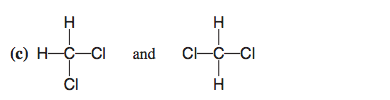# Problem: Consider each pair of structural formulas that follow and state whether the two formulas represent the same compound, whether they represent different compounds that are constitutional isomers of each other, or whether they represent different compounds that are not isomeric.

###### FREE Expert Solution
98% (487 ratings)
###### Problem Details

Consider each pair of structural formulas that follow and state whether the two formulas represent the same compound, whether they represent different compounds that are constitutional isomers of each other, or whether they represent different compounds that are not isomeric.Frequently Asked Questions

What scientific concept do you need to know in order to solve this problem?

Our tutors have indicated that to solve this problem you will need to apply the Constitutional Isomers concept. You can view video lessons to learn Constitutional Isomers. Or if you need more Constitutional Isomers practice, you can also practice Constitutional Isomers practice problems.

What is the difficulty of this problem?

Our tutors rated the difficulty ofConsider each pair of structural formulas that follow and st...as low difficulty.

How long does this problem take to solve?

Our expert Organic tutor, Jonathan took 1 minute undefined to solve this problem. You can follow their steps in the video explanation above.

What textbook is this problem found in?

Our data indicates that this problem or a close variation was asked in Organic Chemistry - Solomons 10th Edition. You can also practice Organic Chemistry - Solomons 10th Edition practice problems.